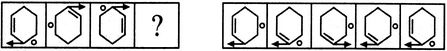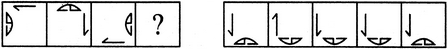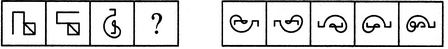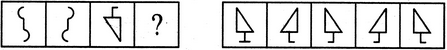# Non Verbal Reasoning - Analogy

Exercise : Analogy - Section 1
Directions to Solve

Each of the following questions consists of two sets of figures. Figures A, B, C and D constitute the Problem Set while figures 1, 2, 3, 4 and 5 constitute the Answer Set. There is a definite relationship between figures A and B. Establish a similar relationship between figures C and D by selecting a suitable figure from the Answer Set that would replace the question mark (?) in fig. (D).

16.

Select a suitable figure from the Answer Figures that would replace the question mark (?).(A)     (B)      (C)     (D)                  (1)      (2)      (3)      (4)      (5)
1
2
3
4
5
Explanation:
The arrow moves four spaces (each space is equal to a side of the hexagon) in a CW direction while the line segment and the circle move two spaces in a CW direction.

17.

Select a suitable figure from the Answer Figures that would replace the question mark (?).(A)     (B)      (C)     (D)                  (1)      (2)      (3)      (4)      (5)
1
2
3
4
5
Explanation:
The line segment moves four spaces ACW and the dot moves three spaces ACW.

18.

Select a suitable figure from the Answer Figures that would replace the question mark (?).(A)     (B)      (C)     (D)                  (1)      (2)      (3)      (4)      (5)
1
2
3
4
5
Explanation:
Both the elements move to the adjacent side in a CW direction; the half-arrow rotates 90o ACW; the semi-circular element rotates 90oACW and gets laterally inverted.

19.

Select a suitable figure from the Answer Figures that would replace the question mark (?).(A)     (B)      (C)     (D)                  (1)      (2)      (3)      (4)      (5)
1
2
3
4
5
Explanation:
The figure rotates 90oACW and gets vertically inverted.

20.

Select a suitable figure from the Answer Figures that would replace the question mark (?).